### Practice Set 1.1 Similarity Class 10th Mathematics Part 2 MHB Solution

Practice Set 1.1
1. Base of a triangle is 9 and height is 5. Base of another triangle is 10 and height is…
2. If figure 1.13 BC ⊥ AB, AD ⊥ AB, BC = 4, AD = 8, then find a (deltaabc)/a (deltaadb)…
3. In adjoining figure 1.14 seg PS seg RQ , seg QT seg PR. If RQ = 6, PS = 6 and PR = 12,…
4. In adjoining figure, AP ⊥ BC, AD || BC, then find A(ΔABC) : A (ΔBCD) n…
5. In adjoining figure PQ BC, AD BC then find following ratios. (i) a (deltapqb)/a…

###### Practice Set 1.1
Question 1.

Base of a triangle is 9 and height is 5. Base of another triangle is 10 and height is 6. Find the ratio of areas of these triangles.

We know that area of triangle =× Base× Height

⇒ Area (triangle 1) =×9× 5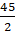⇒ Area (triangle 2) =×10× 6

= 30

∴ the ratio of areas of these triangles will be =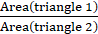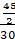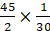Question 2.

If figure 1.13 BC ⊥ AB, AD ⊥ AB, BC = 4, AD = 8, then find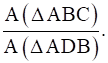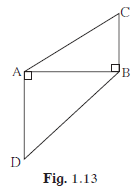Here,ΔABC and ΔADB has common Base.

∴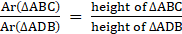(PROPERTY: Areas of triangles with equal bases are proportional to their corresponding heights.)

⇒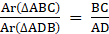Question 3.

In adjoining figure 1.14 seg PS ⊥ seg RQ , seg QT ⊥ seg PR. If RQ = 6, PS = 6 and PR = 12, then find QT.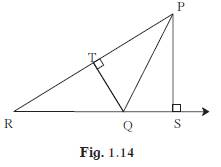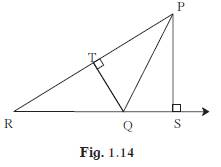Considering, Area of (ΔPQR) with base QR

⇒ PS will be the Height

Now, consider the Area of (ΔPQR) with base PR

⇒ QT will be the Height

∵ , the triangle is the same

⇒ the area will be the same irrespective of the base taken.

And we know that area of triangle =× Base× Height

⇒×QR×PS×PR×QT

⇒×6×6×12×QT

⇒ QT = 3

Question 4.

In adjoining figure, AP ⊥ BC, AD || BC, then find A(ΔABC) : A (ΔBCD)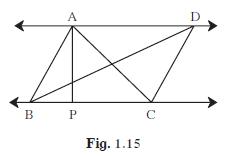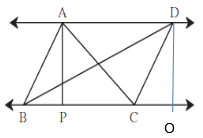We can re-draw the fig.1.15(as shown above) where we add DO

which will be height of ΔBCD.

Now,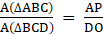(PROPERTY: Areas of triangles with equal bases are proportional to their corresponding heights.)⇒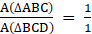(∵ the distance between the two parallel lines is always equal ⇒ AP = DO)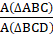= 1:1

Question 5.

In adjoining figure PQ ⊥ BC, AD ⊥ BC then find following ratios.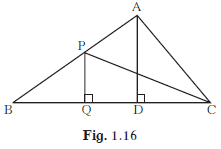(i)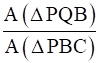(ii)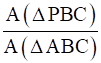(iii)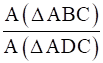(iv)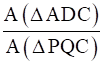We know that area of triangle =× Base× Height

(i)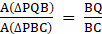(PROPERTY:Areas of triangles with equal heights are proportional to their corresponding bases.)

(ii)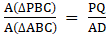(PROPERTY: Areas of triangles with equal bases are proportional to their corresponding heights.)

(iii)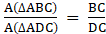(PROPERTY:Areas of triangles with equal heights are proportional to their corresponding bases.)

(iv)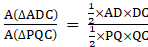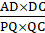## PDF FILE TO YOUR EMAIL IMMEDIATELY PURCHASE NOTES & PAPER SOLUTION. @ Rs. 50/- each (GST extra)

SUBJECTS

HINDI ENTIRE PAPER SOLUTION

MARATHI PAPER SOLUTION
SSC MATHS I PAPER SOLUTION
SSC MATHS II PAPER SOLUTION
SSC SCIENCE I PAPER SOLUTION
SSC SCIENCE II PAPER SOLUTION
SSC ENGLISH PAPER SOLUTION
SSC & HSC ENGLISH WRITING SKILL
HSC ACCOUNTS NOTES
HSC OCM NOTES
HSC ECONOMICS NOTES
HSC SECRETARIAL PRACTICE NOTES

2019 Board Paper Solution

HSC ENGLISH SET A 2019 21st February, 2019

HSC ENGLISH SET B 2019 21st February, 2019

HSC ENGLISH SET C 2019 21st February, 2019

HSC ENGLISH SET D 2019 21st February, 2019

SECRETARIAL PRACTICE (S.P) 2019 25th February, 2019

HSC XII PHYSICS 2019 25th February, 2019

CHEMISTRY XII HSC SOLUTION 27th, February, 2019

OCM PAPER SOLUTION 2019 27th, February, 2019

HSC MATHS PAPER SOLUTION COMMERCE, 2nd March, 2019

HSC MATHS PAPER SOLUTION SCIENCE 2nd, March, 2019

SSC ENGLISH STD 10 5TH MARCH, 2019.

HSC XII ACCOUNTS 2019 6th March, 2019

HSC XII BIOLOGY 2019 6TH March, 2019

HSC XII ECONOMICS 9Th March 2019

SSC Maths I March 2019 Solution 10th Standard11th, March, 2019

SSC MATHS II MARCH 2019 SOLUTION 10TH STD.13th March, 2019

SSC SCIENCE I MARCH 2019 SOLUTION 10TH STD. 15th March, 2019.

SSC SCIENCE II MARCH 2019 SOLUTION 10TH STD. 18th March, 2019.

SSC SOCIAL SCIENCE I MARCH 2019 SOLUTION20th March, 2019

SSC SOCIAL SCIENCE II MARCH 2019 SOLUTION, 22nd March, 2019

XII CBSE - BOARD - MARCH - 2019 ENGLISH - QP + SOLUTIONS, 2nd March, 2019

HSC Maharashtra Board Papers 2020

(Std 12th English Medium)

HSC ECONOMICS MARCH 2020

HSC OCM MARCH 2020

HSC ACCOUNTS MARCH 2020

HSC S.P. MARCH 2020

HSC ENGLISH MARCH 2020

HSC HINDI MARCH 2020

HSC MARATHI MARCH 2020

HSC MATHS MARCH 2020

SSC Maharashtra Board Papers 2020

(Std 10th English Medium)

English MARCH 2020

HindI MARCH 2020

Hindi (Composite) MARCH 2020

Marathi MARCH 2020

Mathematics (Paper 1) MARCH 2020

Mathematics (Paper 2) MARCH 2020

Sanskrit MARCH 2020

Important-formula

THANKS